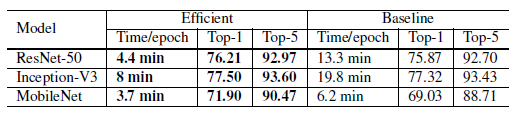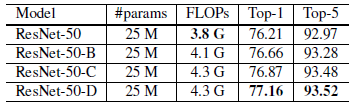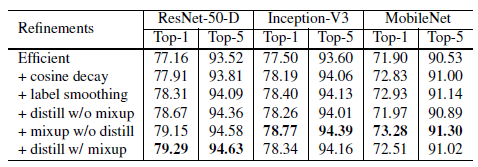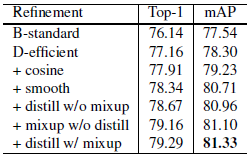# Review — ResNet-D: Bag of Tricks for Image Classification with Convolutional Neural Networks

## ResNet-D, ResNet Using Bag of Tricks, Outperforms ResNeXt, etc.

Bag of Tricks for Image Classification with Convolutional Neural Networks
Bag of Tricks, ResNet-D, by Amazon Web Services
2019 CVPR, Over 700 Citations (Sik-Ho Tsang @ Medium)
Image Classification, Residual Network, ResNet

• Bag of Tricks are applied to improve ResNet: More efficient training, few model tweaks, and some training refinements.
• Some techniques are proposed from previous papers. In this paper, authors try to group all the techniques to boost ResNet performance.

# Outline

1. Baseline Training
2. Efficient Training
3. Model Tweaks
4. Training Refinement
5. Transfer Learning Results

# 1. Baseline Training

• b images are sampled for each batch. It stops after K passes through the dataset.
1. Randomly sample an image and decode it into 32-bit floating point raw pixel values in [0, 255].
2. Randomly crop a rectangular region whose aspect ratio is randomly sampled in [3/4, 4/3] and area randomly sampled in [8%, 100%], then resize the cropped region into a 224×224 square image.
3. Flip horizontally with 0.5 probability.
4. Scale hue, saturation, and brightness with coefficients uniformly drawn from [0.6, 1.4].
5. Add PCA noise with a coefficient sampled from a normal distribution N(0, 0.1).
6. Normalize RGB channels by subtracting 123.68, 116.779, 103.939 and dividing by 58.393, 57.12, 57.375, respectively.
• Nesterov Accelerated Gradient (NAG) descent  is used for training. Each model is trained for 120 epochs on 8 Nvidia V100 GPUs with a total batch size of 256.

ResNet-50 results are slightly better than the reference results, while the baseline Inception-v3 and MobileNetV1 are slightly lower in accuracy due to different training procedure.

# 2. Efficient Training

• Large-batch training and low-precision training are proposed.

## 2.1.1. Linear Scaling Learning Rate

• Increasing the batch size does not change the expectation of the stochastic gradient but reduces its variance. In other words, a large batch size reduces the noise in the gradient, so the learning rate may be increased to make a larger progress.
• Authors follow ResNet to choose 0.1 as the initial learning rate for batch size 256, then when changing to a larger batch size b, the initial learning rate is increased to 0.1×b/256.

## 2.1.2. Learning Rate Warmup

• At the beginning of the training, all parameters are typically random values and therefore far away from the final solution. Using a too large learning rate may result in numerical instability.

In the warmup heuristic, a small learning rate is used at the beginning and then the initial learning rate is switched back later on.

• The first m batches (e.g. 5 data epochs) are used to warm up.

## 2.1.3. Zero γ

• γ=0 is initialized for all BN layers that sit at the end of a residual block. Therefore, all residual blocks just return their inputs, mimics network that has less number of layers and is easier to train at the initial stage.

## 2.1.4. No Bias Decay

• The weight decay is applied to the weights in convolution and fully-connected layers. Other parameters, including the biases and and in BN layers, are left unregularized.

## 2.2. Low-Precision TrainingComparison of the training time and validation accuracy for ResNet-50 between the baseline (BS=256 with FP32) and a more hardware efficient setting (BS=1024 with FP16).
• Neural networks are commonly trained with 32-bit floating point (FP32) precision.
• New hardware, however, may have enhanced arithmetic logic unit for lower precision data types. For example, the previously mentioned Nvidia V100 offers 14 TFLOPS in FP32 but over 100 TFLOPS in FP16.
• As in the above table, the overall training speed is accelerated by 2 to 3 times after switching from FP32 to FP16 on V100.

The model trained with 1024 batch size and FP16 even slightly increased 0.5% top-1 accuracy compared to the baseline model.

Increasing batch size from 256 to 1024 by linear scaling learning rate alone leads to a 0.9% decrease of the top-1 accuracy while stacking the rest three heuristics bridges the gap.

Switching from FP32 to FP16 at the end of training does not affect the accuracy.

# 3. Model Tweaks

• A model tweak is a minor adjustment to the network architecture.
• Such a tweak often barely changes the computational complexity.

## 3.1. ResNet Baseline Architecture

• The input stem has a 7×7 convolution with a stride of 2, followed by a 3×3 max pooling layer also with a stride of 2.
• In the residual block using bottleneck, Path A has three convolutions, whose kernel sizes are 1×1, 3×3 and 1×1, respectively. The first convolution has a stride of 2 to halve the input width and height.
• Some tweaks are done for above parts.

## 3.2. ResNet Tweaks

• Two popular ResNet tweaks are revisited, called ResNet-B and ResNet-C, respectively. A new model tweak ResNet-D is proposed afterwards.

## 3.2.1. ResNet-B

• This tweak first appeared in a Torch implementation.
• ResNet-B switches the strides size of the first two convolutions in path A, so no information is ignored.

## 3.2.2. ResNet-C

• This tweak was proposed in Inception-v3.
• This tweak replacing the 7×7 convolution in the input stem with three conservative 3×3 convolutions

## 3.2.3. ResNet-D

• Empirically, it is found that adding a 2×2 average pooling layer with a stride of 2 before the convolution, whose stride is changed to 1, works well in practice and impacts the computational cost little.Compare ResNet-50 with three model tweaks on model size, FLOPs and ImageNet validation accuracy

With the batch size is 1024 and precision is FP16 used, by using ALL three tweaks, ResNet-50-D improves ResNet-50 by 1%.

# 4. Training Refinements

## 4.1. Cosine Learning Rate Decay

• ResNet decreases rate at 0.1 for every 30 epochs, which called “step decay”. Inception-v3 decreases rate at 0.94 for every two epochs.
• Assume the total number of batches is T (the warmup stage is ignored), then at batch t, the learning rate ηt is computed as:
• where η is the initial learning rate. This is called “cosine” decay.

As can be seen, the cosine decay decreases the learning rate slowly at the beginning, and then becomes almost linear decreasing in the middle, and slows down again at the end, which potentially improves the training progress.

## 4.2. Label Smoothing

• The idea of label smoothing is proposed by Inception-v3, as regularization.
• The purpose of label smoothing is to prevent the largest logit from becoming much larger than all others:
• qi is the modified ground-truth one-hot vector by label smoothing:
• where ε is 0.1 which is a hyperparameter and K is 1000 which is the number of classes for ImageNet.

## 4.3. Knowledge Distillation

• During training, a Distillation loss is added to penalize the difference between the softmax outputs from the teacher model and the learner model.
• where T=20 is the temperature hyper-parameter.
• One example is using a ResNet-152-D as the teacher model to help training ResNet-50.

## 4.4. mixup Training

• In mixup, each time, two examples (xi, yi) and (xj, yj) are randomly sampled. Then a new example is formed by a weighted linear interpolation of these two examples:
• In mixup training, only the new example (^x, ^y) is used.The validation accuracies on ImageNet for stacking training refinements one by one

By stacking cosine decay, label smoothing and mixup, ResNet, Inception-v3 and MobileNetV1 models are steadily improved.

Distillation works well on ResNet, however, it does not work well on Inception-v3 and MobileNetV1.

# 5. Transfer Learning Results

## 5.1. Place365 as Pretraining

• To support the tricks to be transferable to other dataset, a ResNet-50-D model on MIT Places365 dataset is trained.
• The refinements improve the top-5 accuracy consistently on both the validation and test set.

## 5.2. Object DetectionFaster R-CNN performance with various pretrained base networks evaluated on Pascal VOC.

In particular, the best base model with accuracy 79.29% on ImageNet leads to the best mAP at 81.33% on VOC, which outperforms the standard model by 4%.

## 5.3. Semantic Segmentation

The cosine learning rate schedule effectively improves the accuracy of the FCN performance, while other refinements provide suboptimal results.

• A potential explanation to the phenomenon is that semantic segmentation predicts in the pixel level. While models trained with label smoothing, Distillation and mixup favor soften labels, pixel-level information may be blurred and degrade overall pixel-level accuracy.

Later on, there are also other papers further improve ResNet, such as ResNet Strikes Back and ResNet-RS. ResNet-RS also uses ResNet-D.

## Reference

[2019 CVPR] [Bag of Tricks, ResNet-D]
Bag of Tricks for Image Classification with Convolutional Neural Networks

## Image Classification

1989–2019 … [Bag of Tricks, ResNet-D] 2020: [Random Erasing (RE)] [SAOL] [AdderNet] [FixEfficientNet] [BiT] [RandAugment] [ImageNet-ReaL] [ciFAIR] [ResNeSt] [Batch Augment, BA]
2021: [Learned Resizer] [Vision Transformer, ViT] [ResNet Strikes Back] [DeiT] [EfficientNetV2] [MLP-Mixer] [T2T-ViT] [Swin Transformer] [CaiT] [ResMLP] [ResNet-RS]

--

--